# Geometry Shaalaa.com Model Set 2 2019-2020 SSC (English Medium) 10th Standard Board Exam Question Paper Solution

Geometry [Shaalaa.com Model Set 2]
Date: March 2020
Duration: 2h

 1
 1.A | MCQs
 1.A.i

Four alternative answers for the following question is given. Choose the correct alternative.

Two circles intersect each other such that each circle passes through the centre of the other. If the distance between their centres is 12, what is the radius of each circle?

6 cm

12 cm

24 cm

can’t say

Concept: Theorem of Touching Circles
Chapter: [0.03] Circle
 1.A.ii

If in ∆DEF and ∆PQR, ∠D ≅ ∠Q, ∠R ≅ ∠E then which of the following statements is false?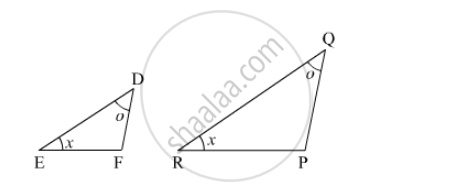$\frac{EF}{PR} = \frac{DF}{PQ}$

$\frac{DE}{PQ} = \frac{EF}{RP}$

$\frac{DE}{QR} = \frac{DF}{PQ}$

$\frac{EF}{RP} = \frac{DE}{QR}$

Concept: Similarity of Triangles
Chapter: [0.01] Similarity
 1.A.iii

Some question and their alternative answer are given. Select the correct alternative.

If a, b, and c are sides of a triangle and a+ b= c2, name the type of triangle.

Obtuse angled triangle

Acute angled triangle

Right-angled triangle

Equilateral triangle

Concept: Right-angled Triangles and Pythagoras Property
Chapter: [0.02] Pythagoras Theorem
 1.A.iv

Select the correct alternative for the following question.

The number of tangents that can be drawn to a circle at a point on the circle is ............... .

3

2

1

0

Concept: Construction of a Tangent to the Circle at a Point on the Circle
Chapter: [0.04] Geometric Constructions
 1.B
 1.B.i

Find the ratio in which point T(–1, 6)divides the line segment joining the points P(–3, 10) and Q(6, –8).

Concept: Division of a Line Segment
Chapter: [0.04] Geometric Constructions [0.05] Co-ordinate Geometry
 1.B.ii

Prove that:

$\cos^2 \theta\left( 1 + \tan^2 \theta \right) = 1$

Concept: Application of Trigonometry
Chapter: [0.06] Trigonometry
 1.B.iii

Find the volume of a cone if the radius of its base is 1.5 cm and its perpendicular height is 5 cm.

Concept: Concept of Surface Area, Volume, and Capacity
Chapter: [0.07] Mensuration
 1.B.iv

Find the coordinates of midpoint of the segment joining the points (22, 20) and (0, 16).

Concept: The Mid-point of a Line Segment (Mid-point Formula)
Chapter: [0.05] Co-ordinate Geometry
 2
 2.A | Solve any 2 of the following
 2.A.i

In the given figure, chord MN and chord RS intersect at point D.
(1) If RD = 15, DS = 4, MD = 8 find DN
(2) If RS = 18, MD = 9, DN = 8 find DS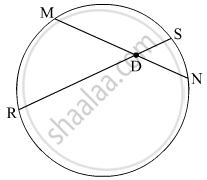Concept: Theorem of External Division of Chords
Chapter: [0.03] Circle
 2.A.ii

In the given figure, if AB || CD || FE then Find x and AE.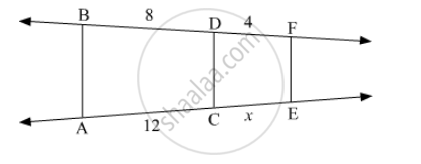Concept: Property of an Angle Bisector of a Triangle
Chapter: [0.01] Similarity
 2.A.iii

If tanθ = 2, find the values of other trigonometric ratios.

Concept: Trigonometric Ratios of Complementary Angles
Chapter: [0.06] Trigonometry
 2.B | Solve any 4 of the following
 2.B.i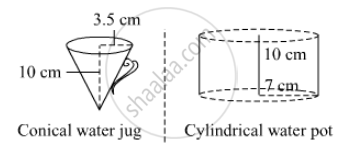Observe the measures of pots In the given figure. How many jugs of water can the cylindrical pot hold?

Concept: Surface Area and Volume of Different Combination of Solid Figures
Chapter: [0.07] Mensuration
 2.B.ii

Determine whether the point is collinear.
A(1, –3), B(2, –5), C(–4, 7)

Concept: Distance Formula
Chapter: [0.05] Co-ordinate Geometry
 2.B.iii

If $\sec\theta = \frac{13}{12}$, find the values of other trigonometric ratios.

Concept: Trigonometric Ratios of Complementary Angles
Chapter: [0.06] Trigonometry
 2.B.iv

In the given figure, altitudes YZ and XT of ∆WXY intersect at P. Prove that,
(1) ▢WZPT is cyclic.
(2) Points X, Z, T, Y are concyclic.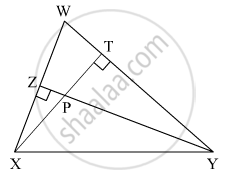Concept: Angle Subtended by the Arc to the Centre
Chapter: [0.03] Circle
 2.B.v

Prove that (sinθ - cosθ + 1)/(sinθ + cosθ - 1) = 1/(secθ - tanθ)

Concept: Application of Trigonometry
Chapter: [0.06] Trigonometry
 3
 3.A | Solve any 1 of the following
 3.A.i

Draw a circle with centre P and radius 3.4 cm. Take point Q at a distance 5.5 cm from the centre. Construct tangents to the circle from point Q.

Concept: To Construct Tangents to a Circle from a Point Outside the Circle.
Chapter: [0.04] Geometric Constructions
 3.A.ii

For finding AB and BC with the help of information given in the figure, complete following activity.

AB = BC ..........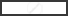$\therefore \angle BAC =$$\therefore AB = BC =$$\times AC$

$=$$\times \sqrt{8}$

$=$$\times 2\sqrt{2}$

=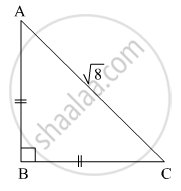Concept: Right-angled Triangles and Pythagoras Property
Chapter: [0.02] Pythagoras Theorem
 3.B | Solve any 2 of the following
 3.B.i

Line l touches a circle with centre O at point P. If radius of the circle is 9 cm, answer the following.
(1) What is d(O, P) = ? Why ?
(2) If d(O, Q) = 8 cm, where does the point Q lie ?
(3) If d(OQ) = 15 cm, How many locations of point Q are line on line l? At what distance will each of them be from point P?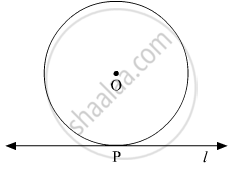Concept: Theorem of Touching Circles
Chapter: [0.03] Circle
 3.B.ii

In the given fig, bisectors of ∠B and ∠C of ∆ABC intersect each other in point X. Line AX intersects side BC in point Y. AB = 5, AC = 4, BC = 6 then find "AX"/"XY".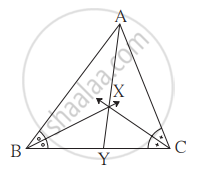Concept: Property of an Angle Bisector of a Triangle
Chapter: [0.01] Similarity
 3.B.iii

Draw a circle with radius 4.1 cm. Construct tangents to the circle from a point at a distance 7.3 cm from the centre.

Concept: To Construct Tangents to a Circle from a Point Outside the Circle.
Chapter: [0.04] Geometric Constructions
 3.B.iv

If A (20, 10), B(0, 20) are given, find the coordinates of the points which divide segment AB into five congruent parts.

Concept: Division of a Line Segment
Chapter: [0.04] Geometric Constructions [0.05] Co-ordinate Geometry
 4 | Solve any 2 of the following
 4.A

A toy is in the form of a cone of radius 3.5 cm mounted on a hemisphere of same radius. The total height of the toy is 15.5 cm. Find the total surface area of the toy [Use π =22/7]

Concept: Surface Area of a Combination of Solids
Chapter:
 4.B

Prove that the perpendicular at the point of contact to the tangent to a circle passes through the centre

Concept: Number of Tangents from a Point on a Circle
Chapter: [0.03] Circle
 4.C

In ∆ABC, B - D - C and BD = 7, BC = 20 then Find following ratio.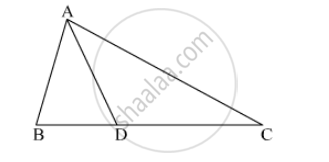"A(∆ ABD)"/"A(∆ ADC)"

Concept: Properties of Ratios of Areas of Two Triangles
Chapter: [0.01] Similarity

In the given fig, XY || seg AC. If 2AX = 3BX and XY = 9. Complete the activity to Find the value of AC.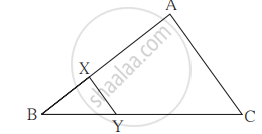Activity : 2AX = 3BX

∴ "AX"/"BX" = square/square

"AX +BX"/"BX" = (square + square)/square ...(by componendo)

"AB"/"BX" = square/square                  ...(I)

ΔBCA ~ ΔBYX                 ... square test of similarity,

∴ "BA"/"BX" = "AC"/"XY"  ...(corresponding sides of similar triangles)

∴ square/square = "AC"/9

∴ AC = square        ...[From(I)]

Concept: Property of Three Parallel Lines and Their Transversals
Chapter: [0.01] Similarity
 5 | Solve any 1 of the following
 5.A

In the given figure, point T is in the interior of rectangle PQRS, Prove that, TS+ TQ= TP+ TR(As shown in the figure, draw seg AB || side SR and A-T-B)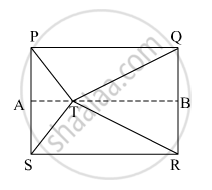Concept: Right-angled Triangles and Pythagoras Property
Chapter: [0.02] Pythagoras Theorem
 5.B

In the given figure, A is the centre of the circle. ∠ABC = 45° and AC = 7√2 cm. Find the area of segment BXC.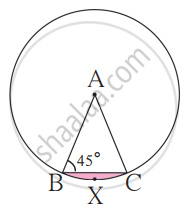Concept: Areas of Sector and Segment of a Circle
Chapter: [0.07] Mensuration

#### Request Question Paper

If you dont find a question paper, kindly write to us

View All Requests

#### Submit Question Paper

Help us maintain new question papers on Shaalaa.com, so we can continue to help students

only jpg, png and pdf files

## Maharashtra State Board previous year question papers 10th Standard Board Exam Geometry with solutions 2019 - 2020

Maharashtra State Board 10th Standard Board Exam Geometry question paper solution is key to score more marks in final exams. Students who have used our past year paper solution have significantly improved in speed and boosted their confidence to solve any question in the examination. Our Maharashtra State Board 10th Standard Board Exam Geometry question paper 2020 serve as a catalyst to prepare for your Geometry board examination.
Previous year Question paper for Maharashtra State Board 10th Standard Board Exam Geometry-2020 is solved by experts. Solved question papers gives you the chance to check yourself after your mock test.
By referring the question paper Solutions for Geometry, you can scale your preparation level and work on your weak areas. It will also help the candidates in developing the time-management skills. Practice makes perfect, and there is no better way to practice than to attempt previous year question paper solutions of Maharashtra State Board 10th Standard Board Exam.

How Maharashtra State Board 10th Standard Board Exam Question Paper solutions Help Students ?
• Question paper solutions for Geometry will helps students to prepare for exam.
• Question paper with answer will boost students confidence in exam time and also give you an idea About the important questions and topics to be prepared for the board exam.
• For finding solution of question papers no need to refer so multiple sources like textbook or guides.